﻿ 框架式防火玻璃隔断的耐火性能研究

# 框架式防火玻璃隔断的耐火性能研究Research on the Fire Resistance Property of Fireproof Glass Partition

Abstract: Fireproof glass as safety glass plays an important role in the building fire. Through the toughed process of glass and heating process of fireproof glass partition in the fire simulated by numerical method, the impact of surface size, width to thickness ratio of glass panel and cooling speeding to the residual stress distribution of toughed glass panel are researched. The quantitative relationship between fire resistance properties of fireproof glass partition and the parameters of glass toughed process are concluded according to the distribution of temperature and stress based on the first strength criterion.

1. 引言

2. 计算理论

${s}_{ij}={\int }_{0}^{t}{G}_{1}\left(\omega -{\omega }^{\prime },\text{\hspace{0.17em}}{T}_{ref}\right)\frac{\text{d}{e}_{ij}}{\text{d}\tau }\text{d}\tau$ (1)

${\sigma }_{kk}={\int }_{0}^{t}{G}_{2}\left(\omega -{\omega }^{\prime }，\text{\hspace{0.17em}}{T}_{ref}\right)\frac{\text{d}}{\text{d}\tau }\left[{\epsilon }_{kk}\left(\tau \right)-{\epsilon }_{th}\left(\tau \right)\right]\text{d}\tau \left(2\right)$ (2)

${e}_{ij}$ 为应变偏量；

${T}_{ref}$ 为参考温度；

${\sigma }_{kk}$${\epsilon }_{kk}$ 为正应力和正应变；

${G}_{1}\left(\omega -{\omega }^{\prime },\text{\hspace{0.17em}}{T}_{ref}\right)$ 为与时间相关的剪切变量；

${G}_{2}\left(\omega -{\omega }^{\prime }，\text{\hspace{0.17em}}{T}_{ref}\right)$ 为与时间相关的体积变量；

${\epsilon }_{th}$ 为热应变； $\omega$${\omega }^{\prime }$ 为时温转换时的减缩时间量。

3. 力学参数取值Table 1. Young’s modulus and Poisson’s ratio at different temperaturesTable 2. Coefficient of thermal conductivity at different temperaturesTable 3. Coefficient of thermal expansion at different temperaturesTable 4. Specific heat capacity at different temperaturesTable 5. Yield stress and plastic strain at different temperatures

4. 玻璃材料破坏准则

4.1. 常温下破坏准则

Griffith断裂理论指出，微观下玻璃开裂由裂纹和缺陷控制，而玻璃材料表面相对于内部布满了大量细微的裂纹和缺陷 。对于玻璃材料而言，材性试验中拉伸玻璃试件时，截面内拉应力均匀分布，随着应力增加玻璃从表面缺陷处开裂然后延申至内部，试件破坏，所以材性试验中所得到的玻璃的极限抗拉强度为表面玻璃材料的极限抗拉强度，低于内部玻璃材料的极限抗拉强度，一般玻璃内部材料强度取为玻璃表面材料强度的1.5倍。本文计算中玻璃极限抗拉强度取为90 MPa，此极限抗拉强度为以往材性试验中测量的一般玻璃表面的极限抗拉强度，内部极限抗拉强度取为135 MPa 。

4.2. 高温下破坏准则

5. 防火玻璃隔断在火灾下数值模拟

5.1. 分析流程

5.2. 模型尺寸与框架参数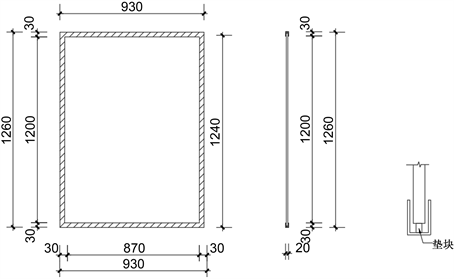Figure 1. The model’s size and structure

5.3. 钢化升温过程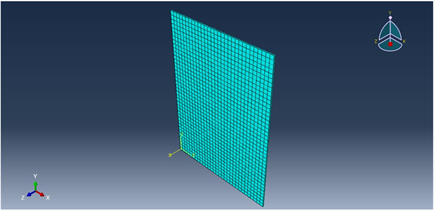Figure 2. Dimension and meshing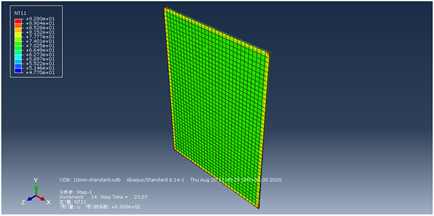Figure 3. Temperature distribution at 23.07 simension and meshing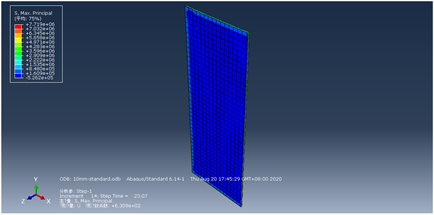Figure 4. The Max principal stress distribution at 23.07 s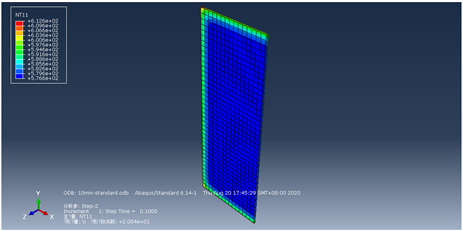Figure 5. Temperature distribution after the heating process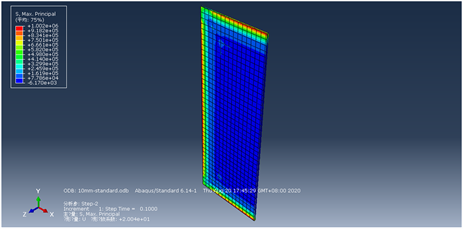Figure 6. The Max principal stress distribution after the heating process

5.4. 钢化冷却过程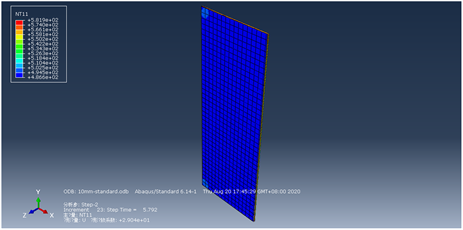Figure 7. Temperature distribution at 5.79 s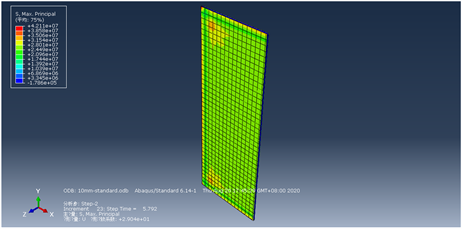Figure 8. The Max principal stress distribution at 5.84 s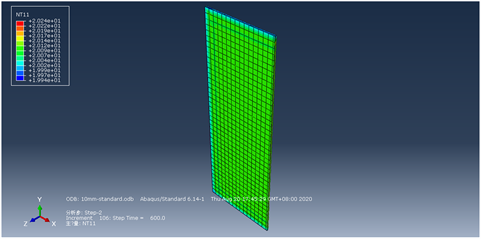Figure 9. Temperature distribution after quenching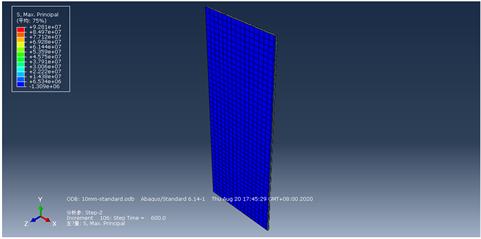Figure 10. The Max principal stress distribution after quenching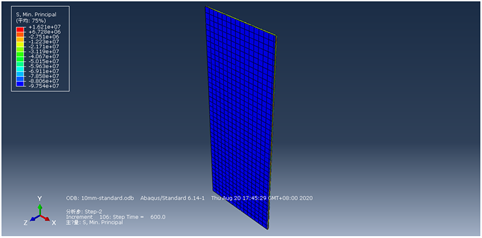Figure 11. The Min principal stress distribution after quenching

5.5. 框架式防火玻璃隔断受火过程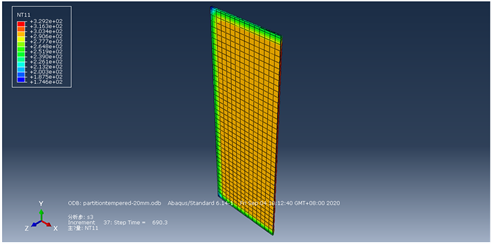Figure 12. Temperature distribution at 692.1 s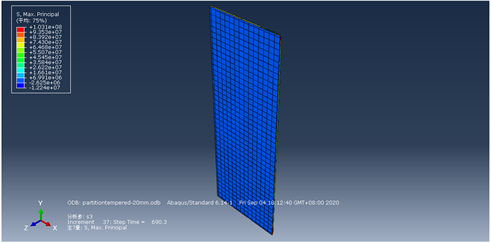Figure 13. The Max principal stress distribution at 692.1 s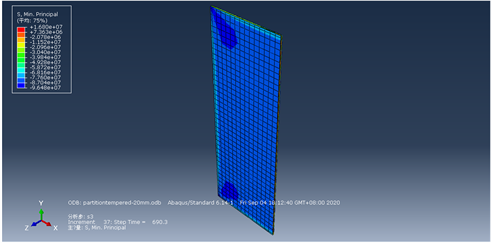Figure 14. The Min principal stress distribution at 692.1 s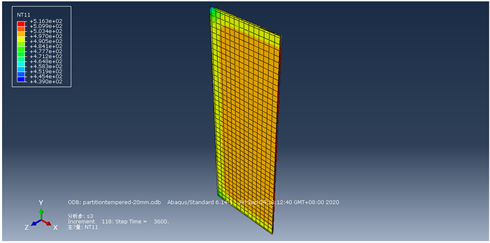Figure 15. Temperature distribution after fire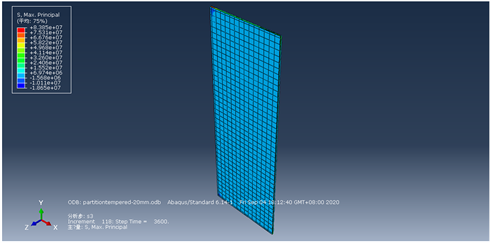Figure 16. The Max principal stress distribution after fire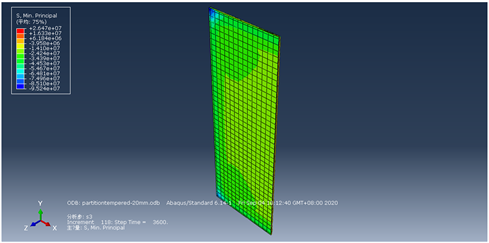Figure 17. The Min principal stress distribution after fire

5.6. 试验案例模拟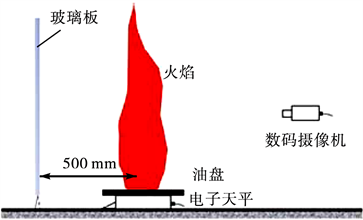Figure 18. Schematic diagram of test apparatus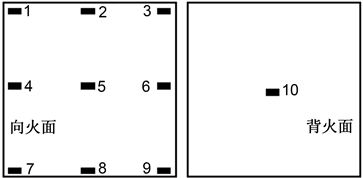Figure 19. Location of patch thermocouple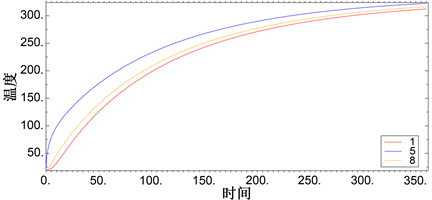Figure 20. Temperature curve of points 1,3,5Figure 21. The Max principal stress distribution at 250 s

6. 防火玻璃隔断参数控制计算

6.1. 玻璃厚度Table 6. Residual stress in different width glass

6.2. 玻璃平面尺寸

Table 7. Residual stress in glasses with different width to thickness ratio

7. 结论

1) 玻璃钢化阶段在升温初期玻璃表面为压应力，内部为拉应力，继续升温玻璃整体温度趋于均匀，热应力逐渐消失；淬火阶段温度逐渐下降，初期玻璃表面为拉应力，内部为压应力，继续降温玻璃表面逐步转变为压应力，内部逐步转表为拉应力，并以此状态保持为永久应力。

2) 由于钢化过程在玻璃表面产生的残余压应力，玻璃边部在整个升温过程中始终处于压应力状态，即在火灾中初始开裂位置不发生在最为脆弱的边部表面，玻璃内部由于裂缝等缺陷较少，强度相对表面较大，玻璃开裂时间将会延后，从而实现延长玻璃隔断耐火时间、提高耐火性能的作用，这证明了防火玻璃隔断的有效性。

3) 不同厚度、平面尺寸玻璃钢化产生残余应力场并不相同，防火玻璃隔断选用玻璃厚度、平面尺寸应根据实际耐火极限需求选择。

4) 通过本模型计算试验案例，第一主应力计算结果预测破坏位置与试验结果相吻合。

 李国强. 钢结构抗火计算与设计[M]. 北京: 中国建筑工业出版社, 1999.

 中华人民共和国国家质量监督检验检疫总局, 中国国家标准化管理委员会. GB15763.1.建筑用安全玻璃, 第一部分: 防火玻璃[M]. 北京: 中国标准出版社, 2009.

 吴再豪. 玻璃钢化冷却过程预控模型与仿真[D]: [硕士学位论文]. 武汉: 武汉科技大学, 2015.

 梁孟羽. 高温下钢化玻璃承重楼板抗弯性能研究[D]: [硕士学位论文]. 北京: 北京建筑大学, 2017.

 周艳艳, 张希艳. 玻璃化学[M]. 北京: 化学工业出版社, 2014.

 陈昊东, 王青松, 孙金华. 火灾环境下玻璃首次破裂时间的模拟预测[J]. 工程力学, 2017(34): 210-213.

 Harada, K., Enomoto, A., Uede, K. and Wakamatsu, T. (1996) An Experimental Study on Glass Cracking and Fallout by Radiant Heat Exposure. Fire Safety Science, 6, 1063-1074.
https://doi.org/10.3801/IAFSS.FSS.6-1063

 王禹, 王青松, 黄柯, 孙金华, 何陵辉, Liew, K.M. 点式安装玻璃幕墙在火灾中的破裂行为[J]. 燃烧科学与技术, 2015(21): 241-247.

Top